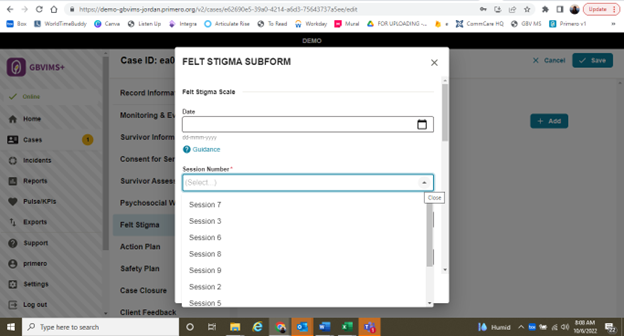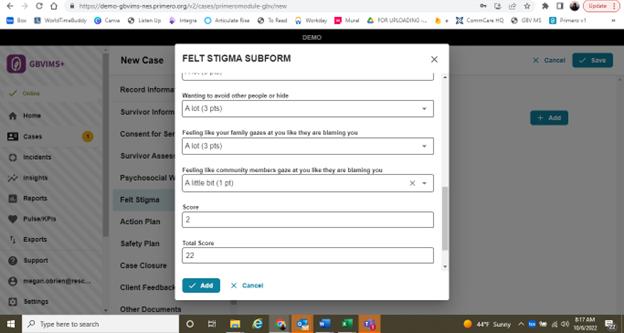# Issues with the Felt Stigma and Psychosocial Wellbeing Forms

I have identified several issues with the Felt Stigma and Psychosocial Wellbeing case forms in GBVIMS+ v2. First, the dropdown menu for session number does not allow me to reorder the numbers so they are sequential (even though they were entered in order when the drop down was created).

Most importantly, the automatic calculations for these forms are still not implemented in Jordan, and they are not working in other instances. The total score works, but the “Score” that is meant to be the average is not calculating correctly.The calculations will not work in Jordan since that was deployed before the work was completed. New implementations going forward from now should work. If this is not the case please let us know which instance

Hi Ian! Thank you for your response! I had several other issues noted in the comment that I want to make sure are clear, too, as they are not specific to Jordan. The automatic calculations are not working in any instance that I can test. The “Score” that is meant to be the average is not calculating correctly. Thank you!

And, what we can do about getting this feature to Jordan since they paid for the development of the calculations? How we can make that happen? Thank you!

We will set up a new instance with the default GBV templates to test the calculations. The options re-ordering within a subform was released with v2.4.3 on 1 September 2022 so the first thing to check is the version you are using. If its greater than v2.4.3 then check the re-ording is working more generally in the instance (in main forms not sub forms for example). If it is not then we will need to work out why

So we have installed the latest templates on the GBV global demo and the calculations seem to work fine. Please can you check that?

Thanks for the update, Ian! I’ve tested the GBV global demo and have found the following:

“Total Score” is working.

“Score” is not working. For example, when I have input the following score (0,1,1), the Score that comes out is 0. The answer should be ((0+1+1)/3) = .67. Or, when I have (1,0,1,0,4,3,1,1,0,2) the Score is reading 1. It should be 1.3.

I hope this testing helps!

The way the average calculation currently works is that it always rounds down and returns the largest integer (not decimal) less than or equal to a given number. In technical terms it calls the `Math.floor()` function.

This means we expect the following results on any calculated number

``````console.log(Math.floor(5.95));
// expected output: 5

console.log(Math.floor(5.05));
// expected output: 5

console.log(Math.floor(5));
// expected output: 5
``````

therefore ((0+1+1)/3) = .67 should return 0 and ((1,0,1,0,4,3,1,1,0,2)/10) = 1.3 should return 1